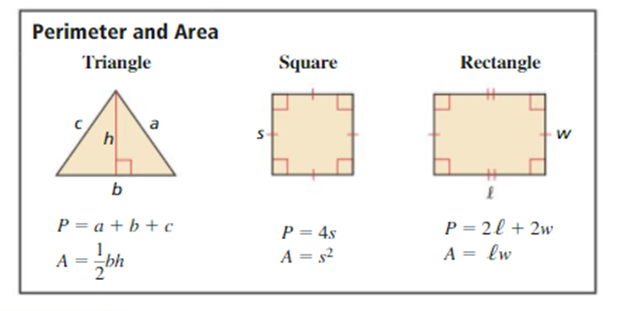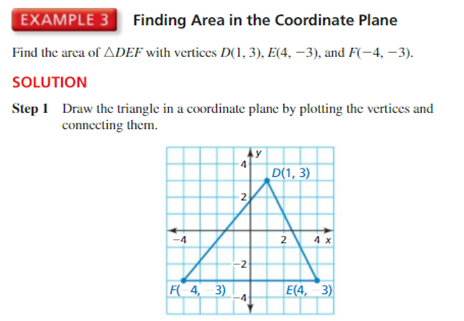# Ch 1.4 Area in the coordinate plane

Author:
Mr. boyce
Topic:
AreaStep 1: determine the distance of the base and height. Base: The base of the triangle goes from point F(-4, -3) to E(4, -3) The side is horizontal so you can either do: /-4-4/ = /-8/ = 8 Or count the graph squares along the line. Height: The height goes from point D(1, 3) straight down to the base at (1,-3) Again you can do: /3- -3/ = /6/ = 6  Or count vertically the graph squares along the line. step 2: input the base and height into the area formula A=1/2bh= ½*8*6= ½*48= 24 Use the graph below to plot (2,3) (4,3) (3,6). Then determine the area of the shape